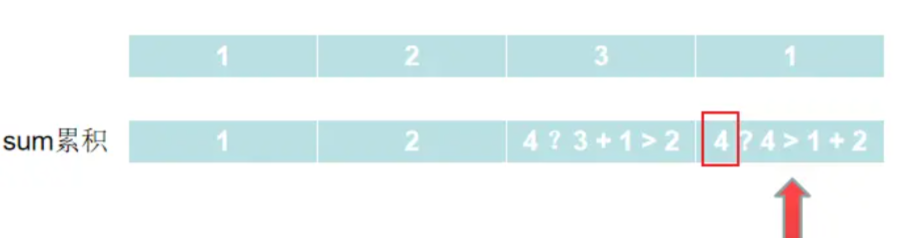# 动态规划：打家劫舍 ⛄

• 2022 年 5 月 27 日
• 本文字数：855 字

阅读完需：约 3 分钟## 打家劫舍

输入：[1,2,3,1]输出：4解释：偷窃 1 号房屋 (金额 = 1) ，然后偷窃 3 号房屋 (金额 = 3)。     偷窃到的最高金额 = 1 + 3 = 4 。

输入：[2,7,9,3,1]输出：12解释：偷窃 1 号房屋 (金额 = 2), 偷窃 3 号房屋 (金额 = 9)，接着偷窃 5 号房屋 (金额 = 1)。     偷窃到的最高金额 = 2 + 9 + 1 = 12 。

[1,2,3,1]为例，得到的sum数组为[1,2,4,4]var rob = function(nums) {    var len = nums.length;    if (len == 0) return 0;    if (len == 1) return nums;    // 定义一个数组，存放从第一家开始每一家能积攒下来的金额    var sum = [];    sum = nums;    sum = Math.max(nums, nums);    for(let i = 2; i < len; i++) {        sum[i] = Math.max(sum[i - 1], sum[i - 2] + nums[i]);    }    return sum[len - 1]};## 评论